# Test: One-Dimensional Steady-state Conduction - 1

## 10 Questions MCQ Test Heat Transfer | Test: One-Dimensional Steady-state Conduction - 1

Description
Attempt Test: One-Dimensional Steady-state Conduction - 1 | 10 questions in 30 minutes | Mock test for Chemical Engineering preparation | Free important questions MCQ to study Heat Transfer for Chemical Engineering Exam | Download free PDF with solutions
QUESTION: 1

Solution:
QUESTION: 2

### Consider the following statements: The Fourier heat conduction equation presumes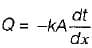1. Steady state conditions 2. Constant value of thermal conductivity 3. Uniform temperature at the wait surfaces 4. One - dimensional heat flow. Which of these statements are correct?

Solution:

k may be constant or vary.

QUESTION: 3

### The heat conducted through a wall of thickness δ is given by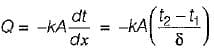Which amongst the following is not valid pertaining to above equation?

Solution:
QUESTION: 4

One end of iron rod of cross-sectional area A and length L is kept in a steambath. After same time steady state conditions prevail and Q unit of heat per second passes through any cross- section of the rod. The heat flow rate will be adversely affected if

Solution: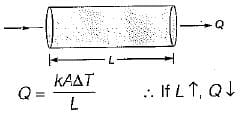QUESTION: 5

Match the List-1 (Coordinate system) with ListII (Steady state governing equation) the following: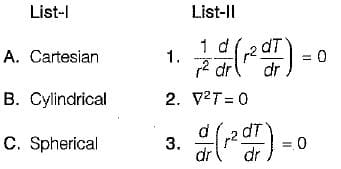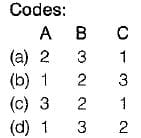Solution:
QUESTION: 6

Temperature distribution in a cylindrical rod with heat generation is given by

Solution:
QUESTION: 7

The Fourier law of heat conduction is valid for

Solution:

While deriving Fourier law of heat conduction some assumptions are made, which are
2. One-directional heat transfer
3. No heat generation
4. Material is homogeneous and isotropic

QUESTION: 8

Fourier law of heat conduction is given by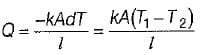where
I = thickness of the slab
dT = temperature difference
In this term l/kA is given by

Solution:

In case of plane slab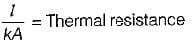QUESTION: 9

Heat transfer by conduction through hollow cylinder is given by

Solution:

From Fourier law of heat conduction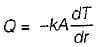For hollow cylinder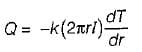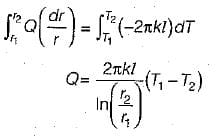QUESTION: 10

Heat transfer by conduction through sphere is given by

Solution:

From Fourier law of heat conduction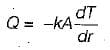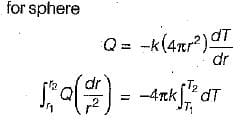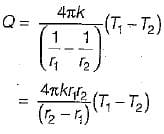Use Code STAYHOME200 and get INR 200 additional OFF Use Coupon Code